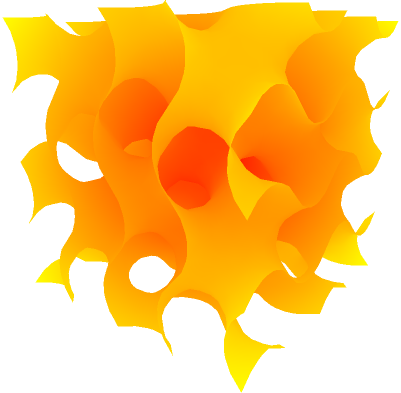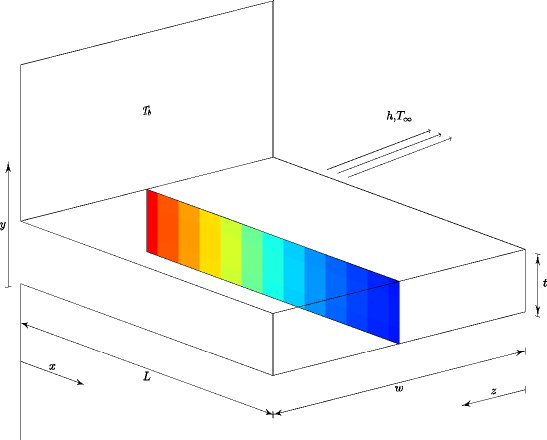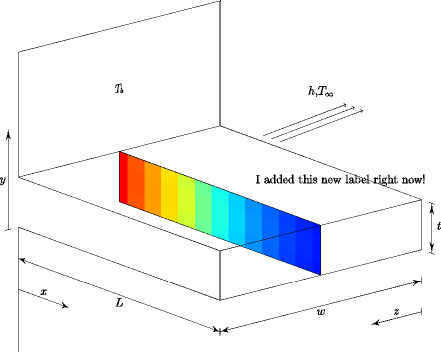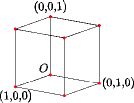### Examples of drawing scientific diagrams with Asymptote in IPython notebook¶

These examples are taken from the gallery on Asymptote's website. We start by installing the asymptote IPython magics remotely from the github repository.

In :
# Install the IPython magic extension
%install_ext http://raw.github.com/azjps/ipython-asymptote/master/asymagic.py

Installed asymagic.py. To use it, type:

In :
# Load the extension


#### Examples¶

In :
%%asy
size(250);

real a=3;
real b=4;
real c=hypot(a,b);

transform ta=shift(c,c)*rotate(-aCos(a/c))*scale(a/c)*shift(-c);
transform tb=shift(0,c)*rotate(aCos(b/c))*scale(b/c);

picture Pythagorean(int n) {
picture pic;
fill(pic,scale(c)*unitsquare,1/(n+1)*green+n/(n+1)*brown);
if(n == 0) return pic;
picture branch=Pythagorean(--n);
return pic;
}


Out:Using the -f --outformat argument, the output format of the image can be changed. By default the image is output as a png. Asymptote may require additional configurations or third party programs (ImageMagick) for additional formats.

In :
%%asy -f png
import graph3;
import palette;
import contour3;
size(400);

real f(real x, real y, real z) {
return cos(x)*sin(y)+cos(y)*sin(z)+cos(z)*sin(x);
}

surface sf=surface(contour3(f,(-2pi,-2pi,-2pi),(2pi,2pi,2pi),12));

currentlight=nolight;

draw(sf,render(merge=true));


Out:A local asymptote code file can also be compiled and displayed if its specified as the argument to an asy file:

In :
!head fin.asy

// See http://asymptote.sourceforge.net/gallery/fin.asy

import three;
import palette;

int N = 26;
real[] C = array(N,0);
real[][] A = new real[N][N];
for(int i = 0; i < N; ++i)
for(int j = 0; j < N; ++j)

In :
%asy fin.asy


Out:We can also interactively append asymptote code to these files:

In :
%%asy fin.asy
label("I added this new label right now!", (2.0,0,0), NE);


Out:To save the intermediate .asy code file and output image, use the -r --root argument.

In :
%%asy --root cube
import three;
currentprojection=orthographic(5,4,2, center=true);

size(5cm);
size3(3cm,5cm,8cm);

draw(unitbox);
dot(unitbox, red);

label("$O$", (0,0,0), NW);
label("(1,0,0)", (1,0,0), S);
label("(0,1,0)", (0,1,0), E);
label("(0,0,1)", (0,0,1), Z);


Out:In :
!head cube.asy

import three;
currentprojection=orthographic(5,4,2, center=true);

size(5cm);
size3(3cm,5cm,8cm);

draw(unitbox);
dot(unitbox, red);

label("$O$", (0,0,0), NW);